ISAR#1
Define, in general terms, what is meant by interferometry.
Interferometry is a method for estimating a topography by comparing phase differences with differences in range between two antennae separated by a known distance and targets.

ISAR#2
Define, in general terms, what is meant by Synthetic Aperture Radar.

ISAR#3
Describe the various components in the diagram below, which represents the key elements involved ISAR: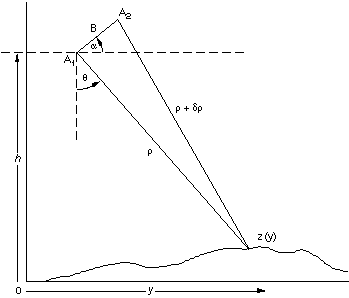What do the quantities y, z,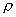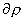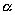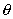and B represent in terms of the process for interferometric synthetic aperture radar?

ISAR#4
Explain the relation,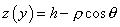.  Why does y not appear on the right hand side if z is a function of y?

ISAR#5
Justify the sequence of equations in detail: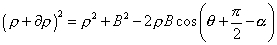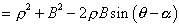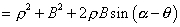and show how these lead to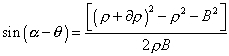ISAR#6
The phase difference f  between the two antennas is directly proportional to, with constant of proportionality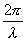.  Explain how this relation leads to the equation,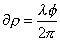, where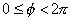.  What doesrepresent in this equation?

ISAR#7
Relate f and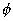in an equation involving a whole number n.   What doand n represent?

At this stage, a technique called "phase unwrapping," is needed to solve for the integer number of wavelengths, n, and obtain the absolute range. Height estimates are averaged over a surface resolution element of the radar image (often termed a picture element or "pixel"), typically tens of meters in diameter.

The goal is get reasonably accurate height estimates.  To get a handle on how accurate these are, it helps to relate the uncertainty in height,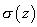, to the uncertainty in the orientation (altitude) angle,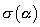.

ISAR#8
Explain why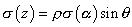.

For a spacecraft system where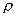is large (at least several hundred kilometers), this last equation shows that the altitude errormust be very small to achieve acceptable height accuracy.

ISAR#9
Show that, for example, to achieve a height accuracy of 2 m from a 500-km-altitude spacecraft with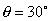, the attitude error must be no more than about 1 arc sec.

For an L-band radar system with B = 1km, this implies a relative position measurement accuracy (i.e., knowledge of the position of one antenna with respect to the other) of about 5 mm. This accuracy is feasible with modern spacecraft-tracking techniques using, for instance, the Global Positioning System (GPS). Errors in the baseline orientation cause errors in absolute elevation, e.g., with respect to the center of mass of the Earth, but the relative heights within the radar swath are largely unaffected. This implies that a few ground control points can be used to calibrate absolute height estimates even in the absence of arc-second attitude information.

Now consider a single-antenna SAR system that revisits the same position and images the same area on the ground after several days or weeks--the so-called "repeat-pass approach."  Assuming there has been no significant change in the surface between acquisition of the two images, we can perform an analysis similar to the dual-antenna case and recover an estimate of topography with essentially the same accuracy. Again, the key is knowledge of the baseline length and orientation (vector B) between the two antenna positions; only in this case, it is the same physical antenna in a different position at a later time.

Estimating topography from repeat-pass observations is obviously more challenging than from simultaneous observations. However, if the aircraft or spacecraft is accurately tracked so that its position and orientation are known as function of Time, the problem is tractable.

ISAR#10
Explain why the analysis is nearly identical to the case illustrated in the diagram except that, since each antenna now acts as both transmitter and receiver, there is a factor of 2 difference in the equation of problem 6,  relating phase and round-trip differential range to account for round-trip distance, so that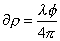.

previous / next